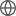Old Web
English
We present the cosmological implications from final measurements of clustering using galaxies, quasars, and Ly$\alpha$ forests from the completed Sloan Digital Sky Survey (SDSS) lineage of experiments in large-scale structure. These experiments, composed of data from SDSS, SDSS-II, BOSS, and eBOSS, offer independent measurements of baryon acoustic oscillation (BAO) measurements of angular-diameter distances and Hubble distances relative to the sound horizon, $r_d$, from eight different samples and six measurements of the growth rate parameter, $f\sigma_8$, from redshift-space distortions (RSD). This composite sample is the most constraining of its kind and allows us to perform a comprehensive assessment of the cosmological model after two decades of dedicated spectroscopic observation. We show that the BAO data alone are able to rule out dark-energy-free models at more than eight standard deviations in an extension to the flat, $\Lambda$CDM model that allows for curvature. When combined with Planck Cosmic Microwave Background (CMB) measurements of temperature and polarization the BAO data provide nearly an order of magnitude improvement on curvature constraints. The RSD measurements indicate a growth rate that is consistent with predictions from Planck primary data and with General Relativity. When combining the results of SDSS BAO and RSD with external data, all multiple-parameter extensions remain consistent with a $\Lambda$CDM model. Regardless of cosmological model, the precision on $\Omega_\Lambda$, $H_0$, and $\sigma_8$, remains at roughly 1\%, showing changes of less than 0.6\% in the central values between models. The inverse distance ladder measurement under a o$w_0w_a$CDM yields $H_0= 68.20 \pm 0.81 \, \rm km\, s^{-1} Mpc^{-1}$, remaining in tension with several direct determination methods. (abridged)